Скачать презентацию Solutions and Concentration Many times in the

a0d5a8f493e6314787644771fb2a76e9.ppt

• Количество слайдов: 29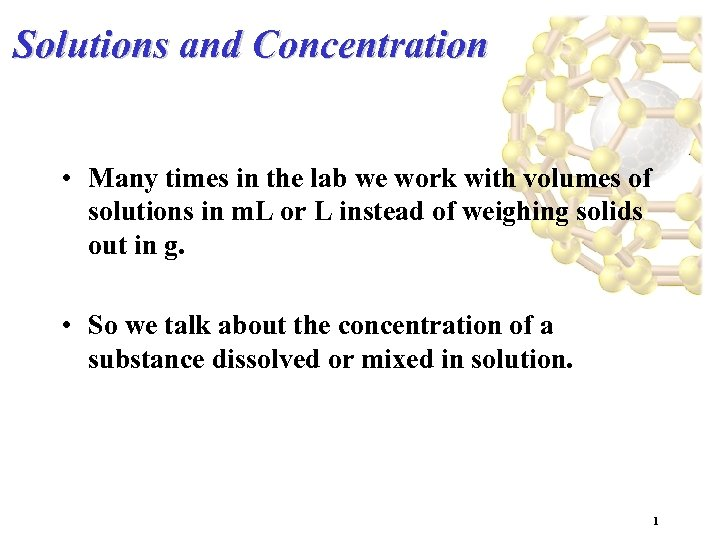Solutions and Concentration • Many times in the lab we work with volumes of solutions in m. L or L instead of weighing solids out in g. • So we talk about the concentration of a substance dissolved or mixed in solution. 1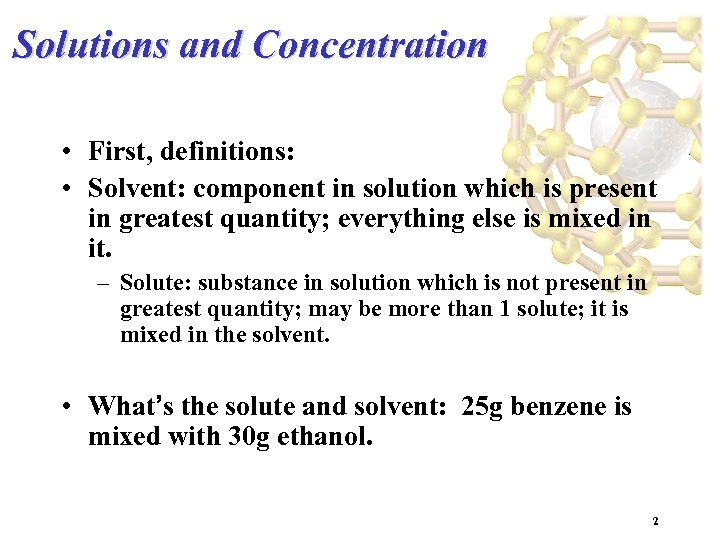Solutions and Concentration • First, definitions: • Solvent: component in solution which is present in greatest quantity; everything else is mixed in it. – Solute: substance in solution which is not present in greatest quantity; may be more than 1 solute; it is mixed in the solvent. • What’s the solute and solvent: 25 g benzene is mixed with 30 g ethanol. 2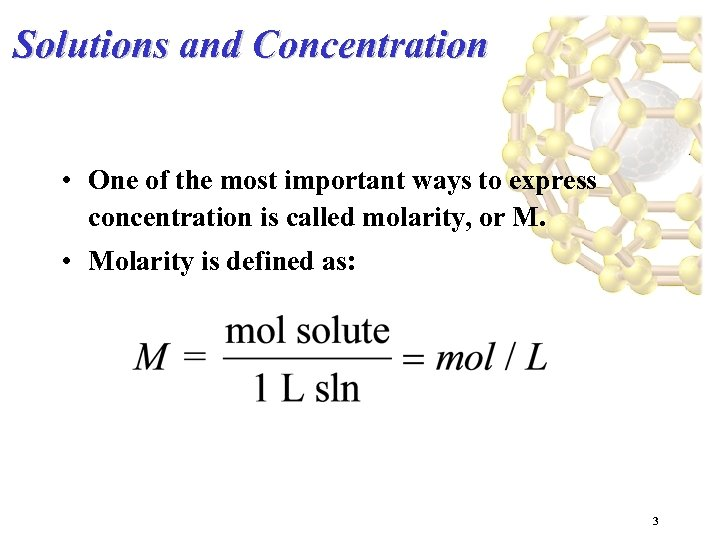Solutions and Concentration • One of the most important ways to express concentration is called molarity, or M. • Molarity is defined as: 3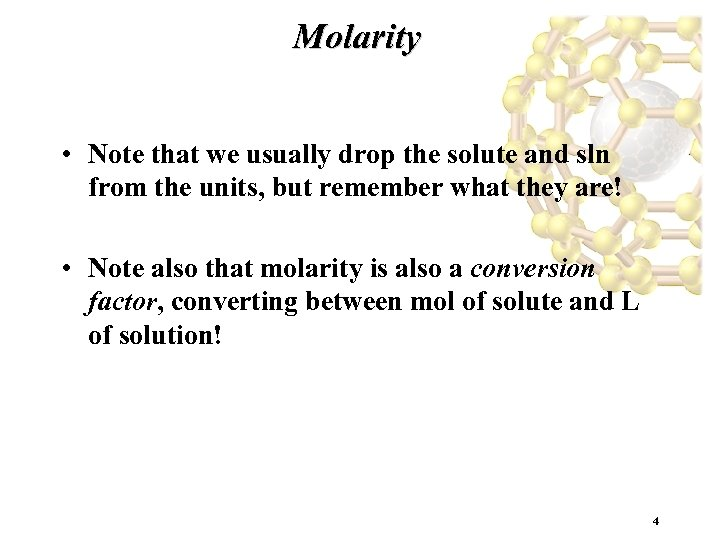Molarity • Note that we usually drop the solute and sln from the units, but remember what they are! • Note also that molarity is also a conversion factor, converting between mol of solute and L of solution! 4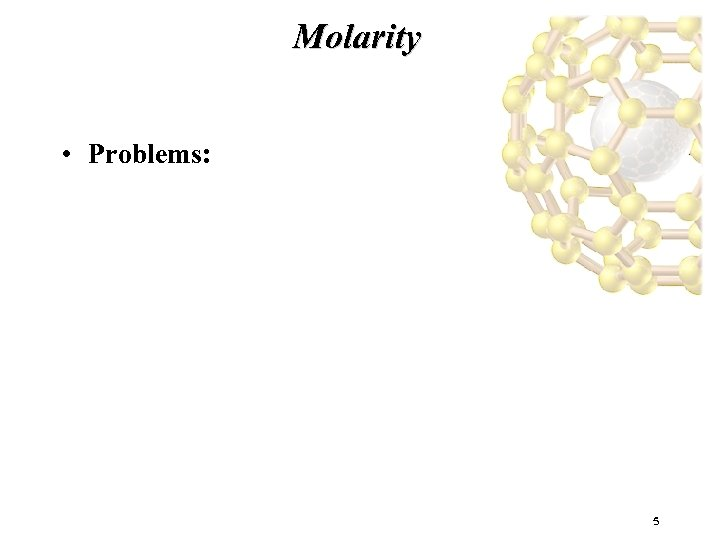Molarity • Problems: 5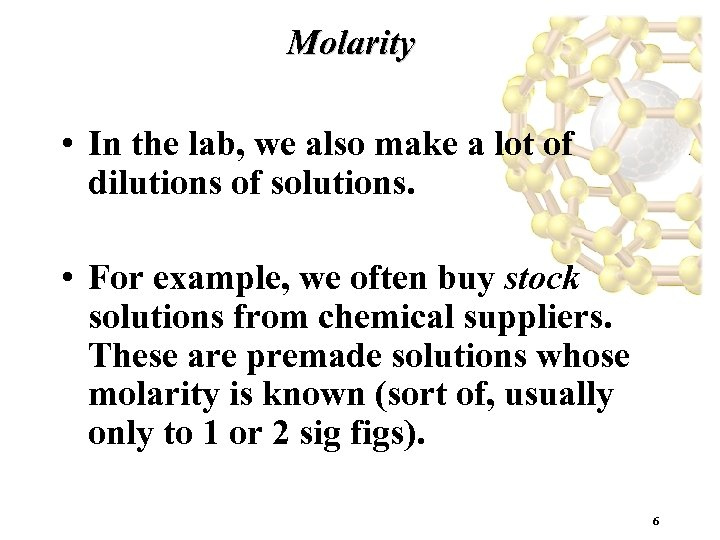Molarity • In the lab, we also make a lot of dilutions of solutions. • For example, we often buy stock solutions from chemical suppliers. These are premade solutions whose molarity is known (sort of, usually only to 1 or 2 sig figs). 6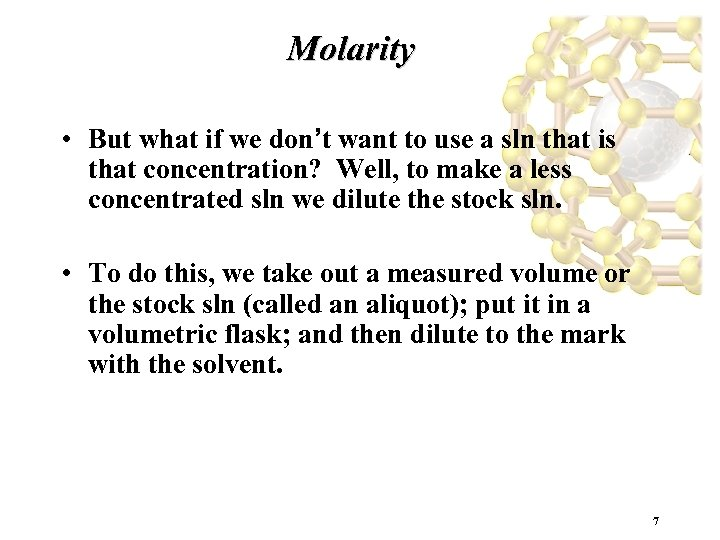Molarity • But what if we don’t want to use a sln that is that concentration? Well, to make a less concentrated sln we dilute the stock sln. • To do this, we take out a measured volume or the stock sln (called an aliquot); put it in a volumetric flask; and then dilute to the mark with the solvent. 7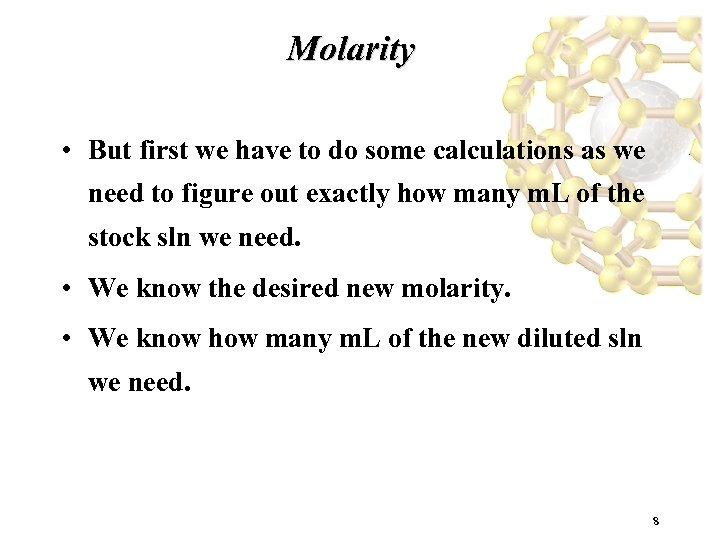Molarity • But first we have to do some calculations as we need to figure out exactly how many m. L of the stock sln we need. • We know the desired new molarity. • We know how many m. L of the new diluted sln we need. 8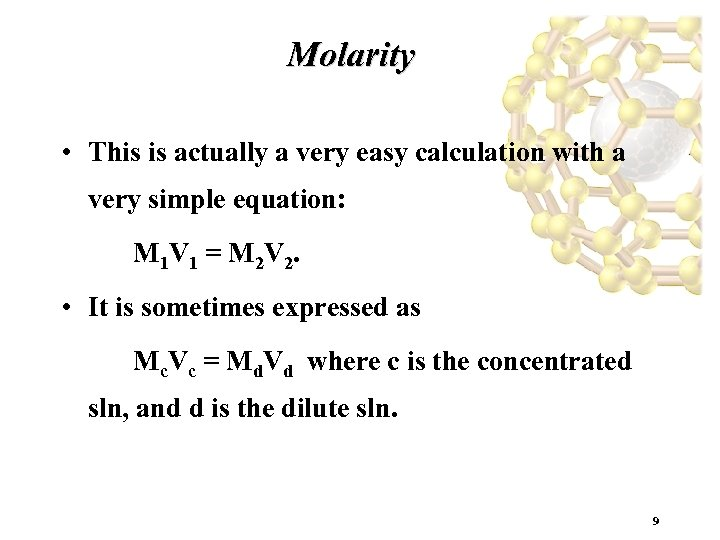Molarity • This is actually a very easy calculation with a very simple equation: M 1 V 1 = M 2 V 2. • It is sometimes expressed as Mc. Vc = Md. Vd where c is the concentrated sln, and d is the dilute sln. 9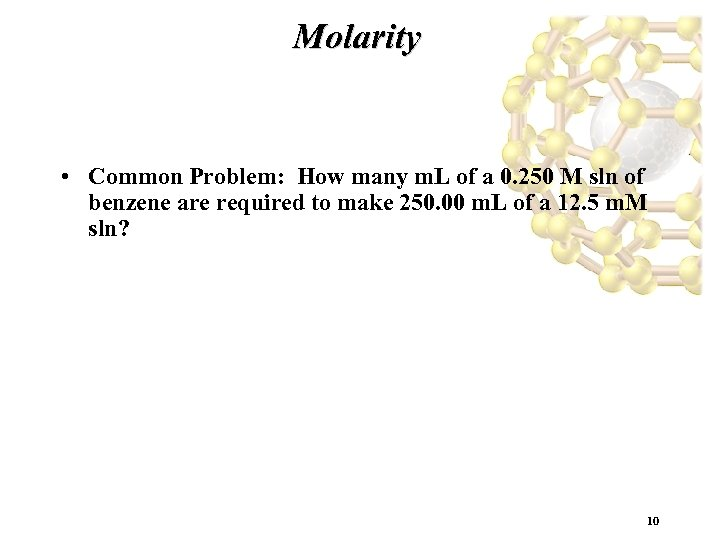Molarity • Common Problem: How many m. L of a 0. 250 M sln of benzene are required to make 250. 00 m. L of a 12. 5 m. M sln? 10Solution Stoichiometry 11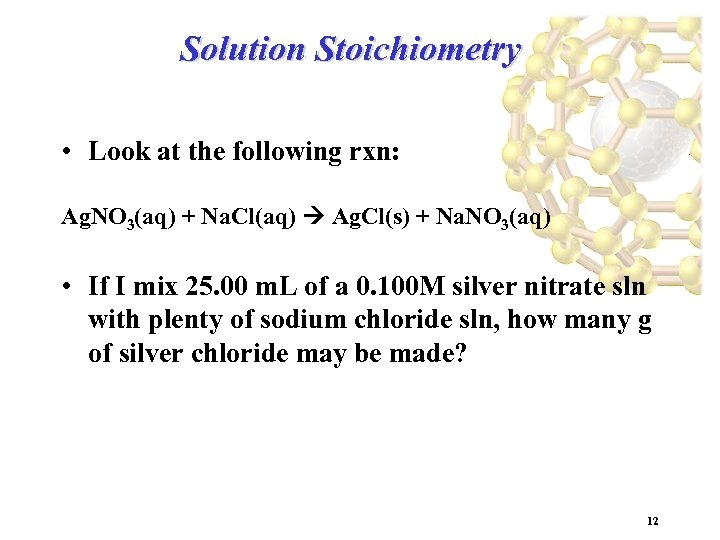Solution Stoichiometry • Look at the following rxn: Ag. NO 3(aq) + Na. Cl(aq) Ag. Cl(s) + Na. NO 3(aq) • If I mix 25. 00 m. L of a 0. 100 M silver nitrate sln with plenty of sodium chloride sln, how many g of silver chloride may be made? 12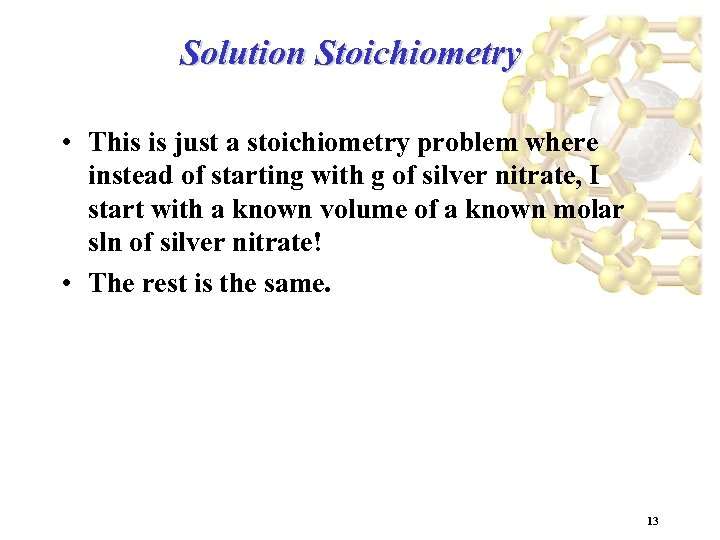Solution Stoichiometry • This is just a stoichiometry problem where instead of starting with g of silver nitrate, I start with a known volume of a known molar sln of silver nitrate! • The rest is the same. 13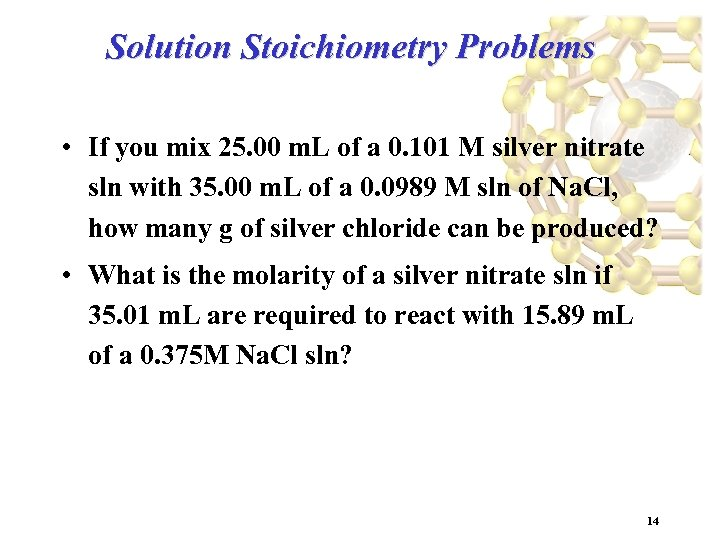Solution Stoichiometry Problems • If you mix 25. 00 m. L of a 0. 101 M silver nitrate sln with 35. 00 m. L of a 0. 0989 M sln of Na. Cl, how many g of silver chloride can be produced? • What is the molarity of a silver nitrate sln if 35. 01 m. L are required to react with 15. 89 m. L of a 0. 375 M Na. Cl sln? 14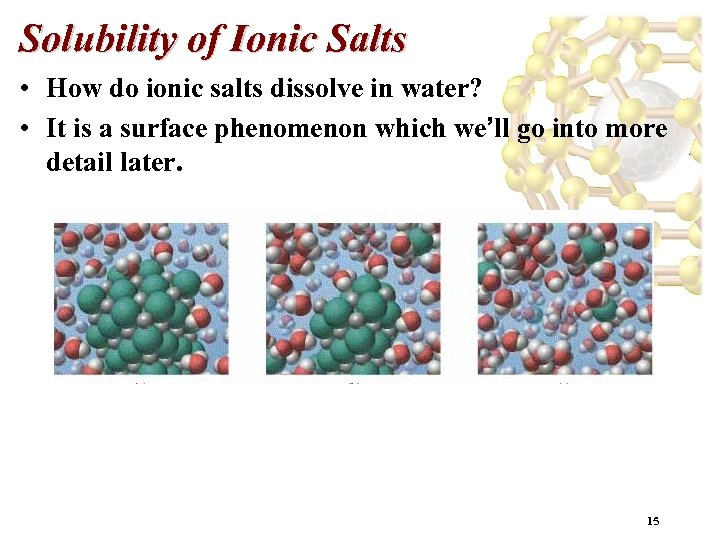Solubility of Ionic Salts • How do ionic salts dissolve in water? • It is a surface phenomenon which we’ll go into more detail later. 15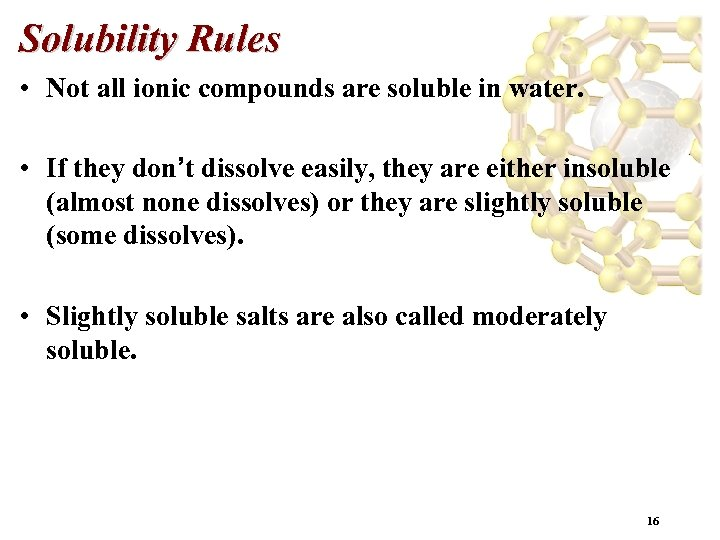Solubility Rules • Not all ionic compounds are soluble in water. • If they don’t dissolve easily, they are either insoluble (almost none dissolves) or they are slightly soluble (some dissolves). • Slightly soluble salts are also called moderately soluble. 16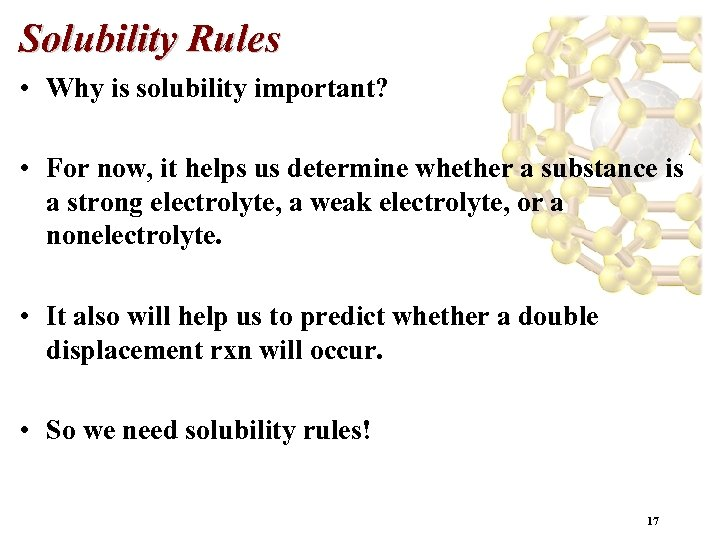Solubility Rules • Why is solubility important? • For now, it helps us determine whether a substance is a strong electrolyte, a weak electrolyte, or a nonelectrolyte. • It also will help us to predict whether a double displacement rxn will occur. • So we need solubility rules! 17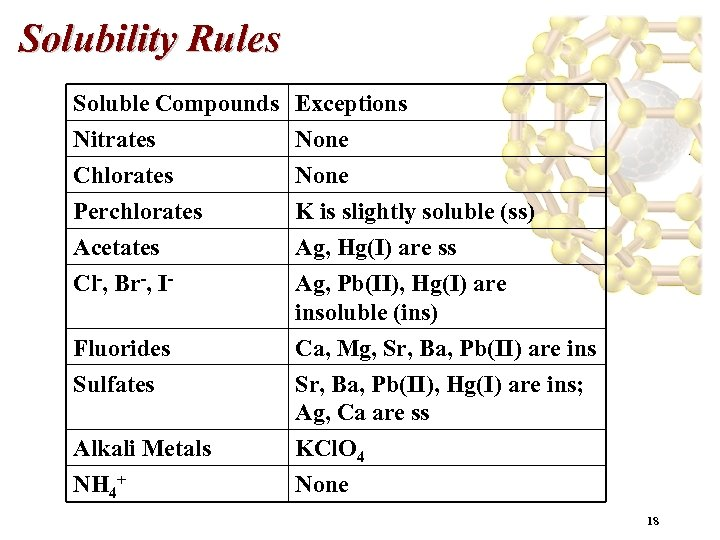Solubility Rules Soluble Compounds Nitrates Chlorates Perchlorates Exceptions None K is slightly soluble (ss) Acetates Cl-, Br-, I- Ag, Hg(I) are ss Ag, Pb(II), Hg(I) are insoluble (ins) Fluorides Sulfates Ca, Mg, Sr, Ba, Pb(II) are ins Sr, Ba, Pb(II), Hg(I) are ins; Ag, Ca are ss KCl. O 4 None Alkali Metals NH 4+ 18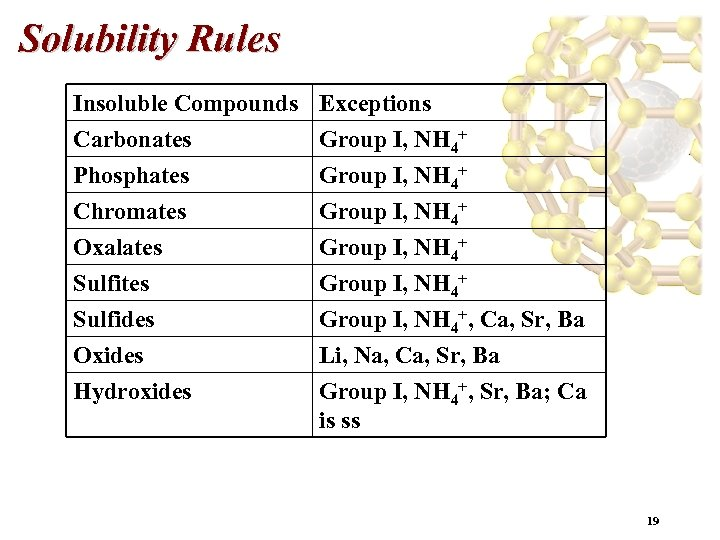Solubility Rules Insoluble Compounds Carbonates Phosphates Chromates Exceptions Group I, NH 4+ Oxalates Sulfides Group I, NH 4+, Ca, Sr, Ba Oxides Hydroxides Li, Na, Ca, Sr, Ba Group I, NH 4+, Sr, Ba; Ca is ss 19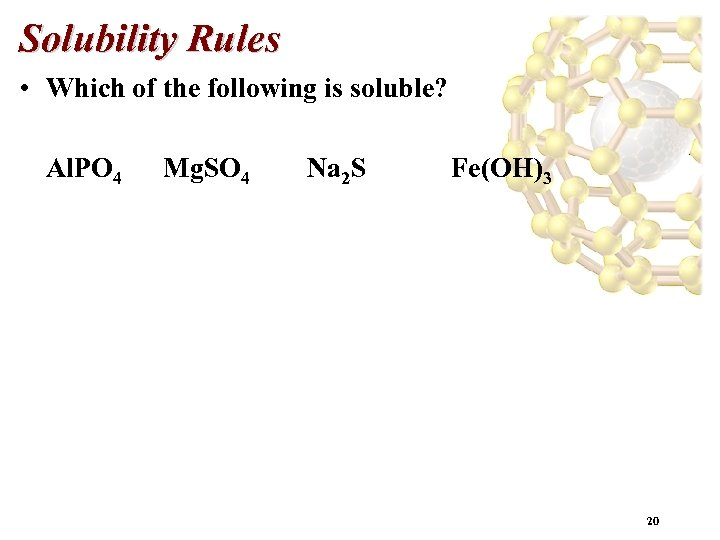Solubility Rules • Which of the following is soluble? Al. PO 4 Mg. SO 4 Na 2 S Fe(OH)3 20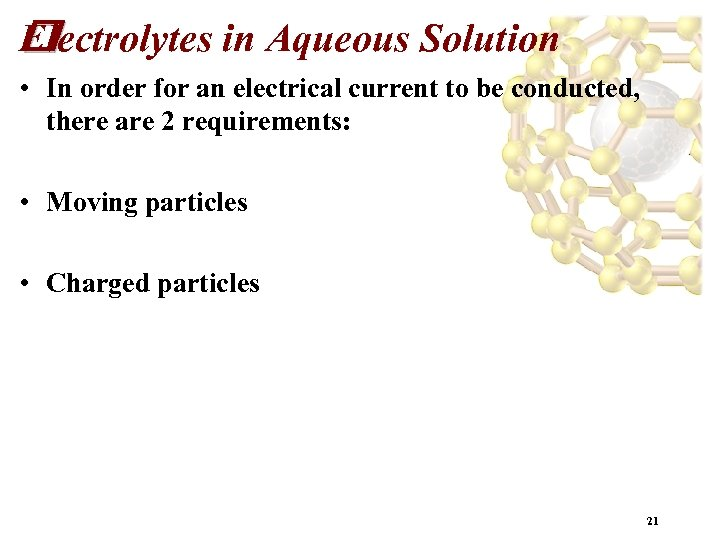Electrolytes in Aqueous Solution • In order for an electrical current to be conducted, there are 2 requirements: • Moving particles • Charged particles 21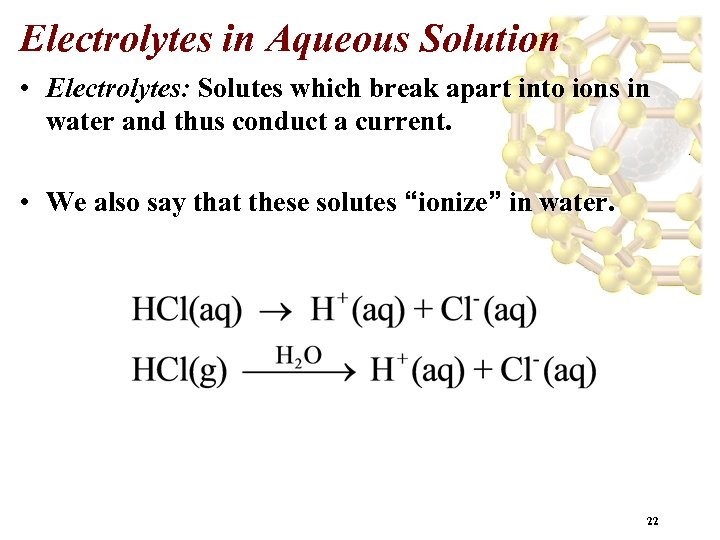Electrolytes in Aqueous Solution • Electrolytes: Solutes which break apart into ions in water and thus conduct a current. • We also say that these solutes “ionize” in water. 22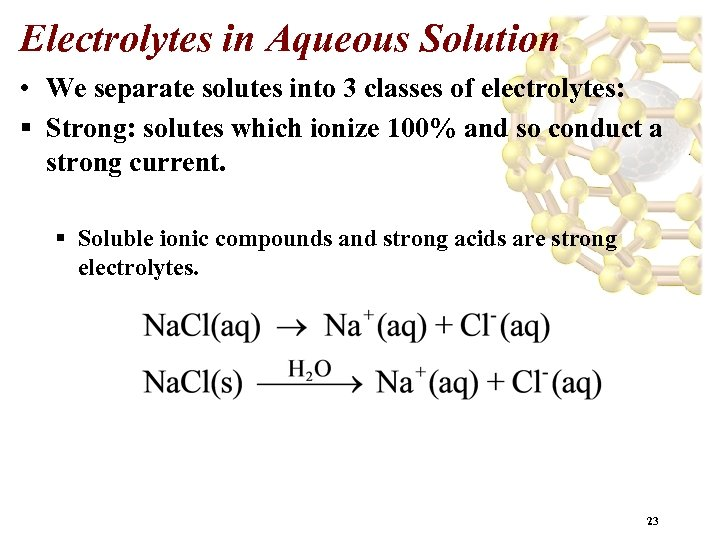Electrolytes in Aqueous Solution • We separate solutes into 3 classes of electrolytes: § Strong: solutes which ionize 100% and so conduct a strong current. § Soluble ionic compounds and strong acids are strong electrolytes. 23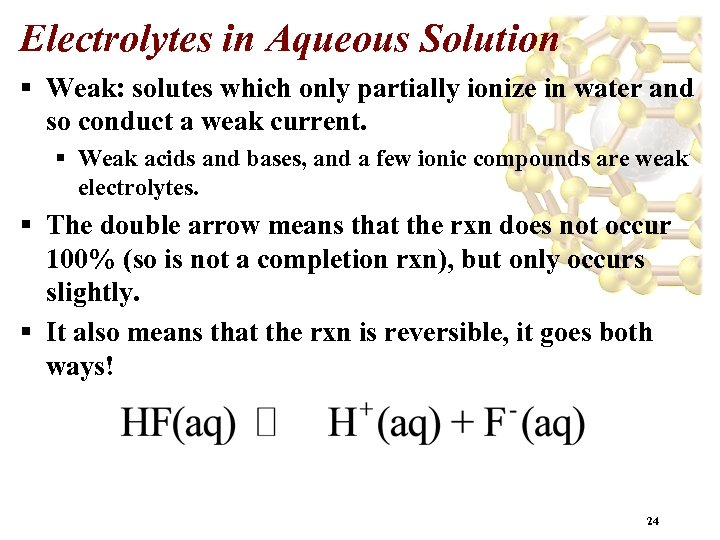Electrolytes in Aqueous Solution § Weak: solutes which only partially ionize in water and so conduct a weak current. § Weak acids and bases, and a few ionic compounds are weak electrolytes. § The double arrow means that the rxn does not occur 100% (so is not a completion rxn), but only occurs slightly. § It also means that the rxn is reversible, it goes both ways! 24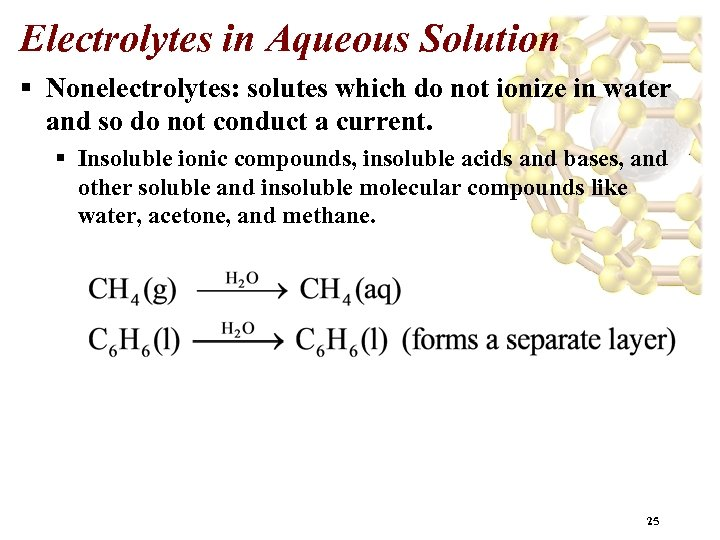Electrolytes in Aqueous Solution § Nonelectrolytes: solutes which do not ionize in water and so do not conduct a current. § Insoluble ionic compounds, insoluble acids and bases, and other soluble and insoluble molecular compounds like water, acetone, and methane. 25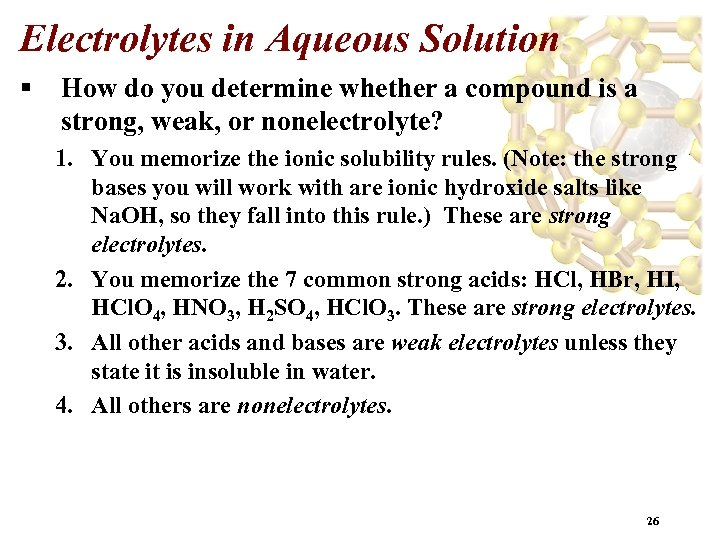Electrolytes in Aqueous Solution § How do you determine whether a compound is a strong, weak, or nonelectrolyte? 1. You memorize the ionic solubility rules. (Note: the strong bases you will work with are ionic hydroxide salts like Na. OH, so they fall into this rule. ) These are strong electrolytes. 2. You memorize the 7 common strong acids: HCl, HBr, HI, HCl. O 4, HNO 3, H 2 SO 4, HCl. O 3. These are strong electrolytes. 3. All other acids and bases are weak electrolytes unless they state it is insoluble in water. 4. All others are nonelectrolytes. 26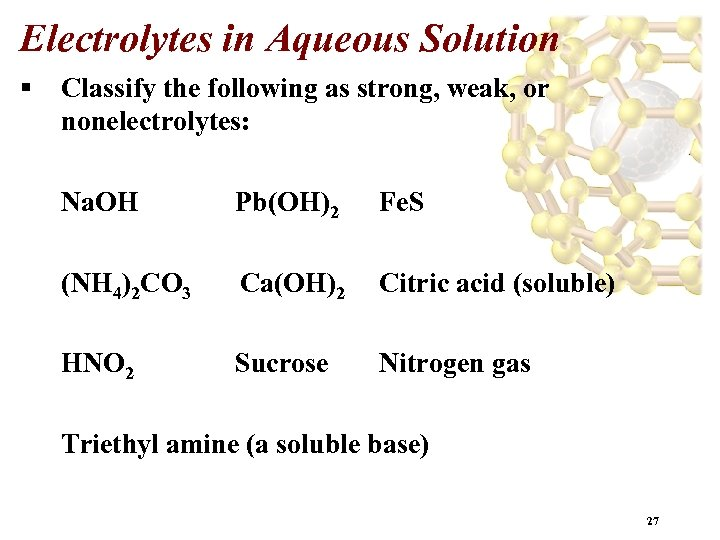Electrolytes in Aqueous Solution § Classify the following as strong, weak, or nonelectrolytes: Na. OH Pb(OH)2 Fe. S (NH 4)2 CO 3 Ca(OH)2 Citric acid (soluble) HNO 2 Sucrose Nitrogen gas Triethyl amine (a soluble base) 27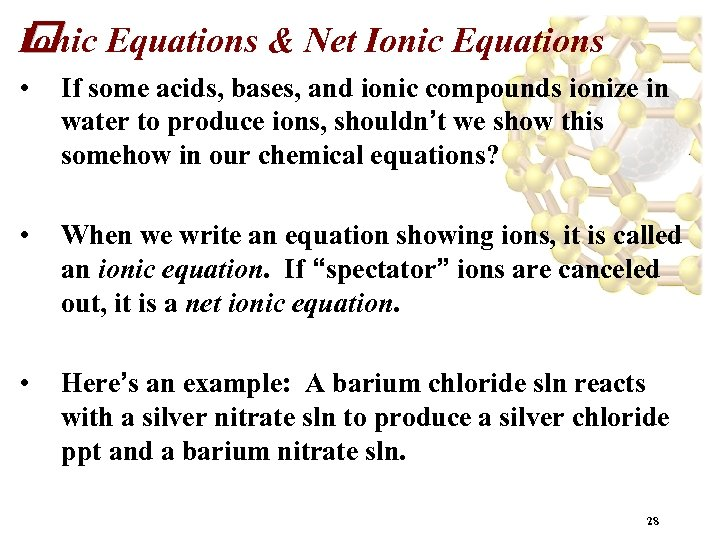Ionic Equations & Net Ionic Equations • If some acids, bases, and ionic compounds ionize in water to produce ions, shouldn’t we show this somehow in our chemical equations? • When we write an equation showing ions, it is called an ionic equation. If “spectator” ions are canceled out, it is a net ionic equation. • Here’s an example: A barium chloride sln reacts with a silver nitrate sln to produce a silver chloride ppt and a barium nitrate sln. 28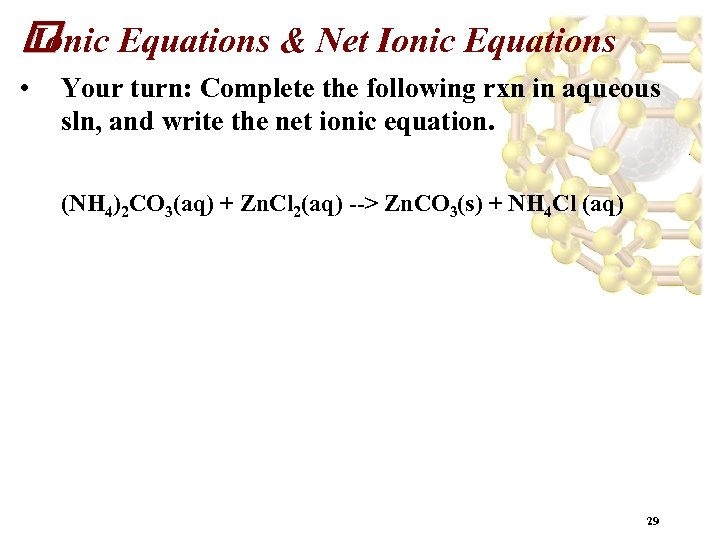Equations & Net Ionic Equations Ionic • Your turn: Complete the following rxn in aqueous sln, and write the net ionic equation. (NH 4)2 CO 3(aq) + Zn. Cl 2(aq) --> Zn. CO 3(s) + NH 4 Cl (aq) 29